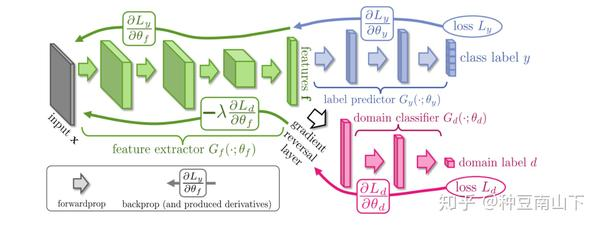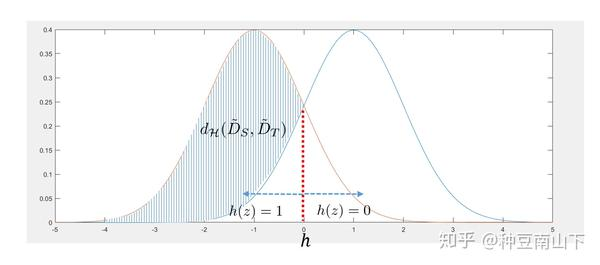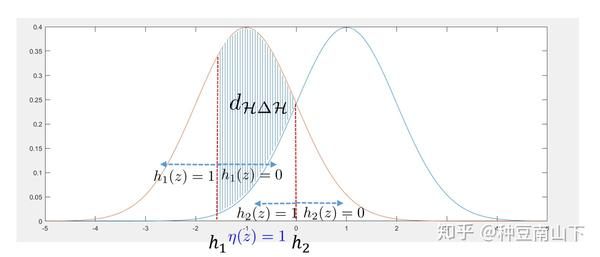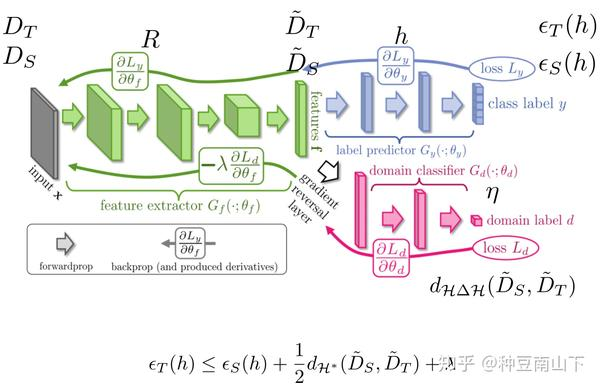# 迁移学习：领域自适应的理论分析

## 背景$Gf$：_将数据映射到feature space，使 $G_y$ 能分辨出source domain数据的label，$G_d$ 分辨不出数据来自source domain还是target domain。

$Gy$ **：_**对feature space的source domain数据进行分类，尽可能分出正确的label。

$Gd$**：_**对feature space的数据进行领域分类，尽量分辨出数据来自于哪一个domain。

## 理论分析

• 问题建立

$\mathcal Z$ 是一个特征空间(feature space)。

$\mathcal D_S$ 是定义在 $\mathcal X$ 上的源域数据分布，$\tilde{\mathcal D}_S$是定义在$\mathcal Z$上的源域特征分布。

$\mathcal D_T$、 $\tilde{\mathcal D}_T$ 一样定义目标域数据分布和特征分布。

$\mathcal R$ : $\mathcal X$ $\rightarrow$ $\mathcal Z$ 是表示函数(representation function)将实例$\mathcal X$映射到 $\mathcal Z$ 上，即上图的 $G_f$ 。

$f$ : $\mathcal X $$\rightarrow {0,1} 是真实的标签函数，是二值函数。我们并不知道f是什么，希望通过训练得到它。 h : \mathcal Z \rightarrow$${0,1}$是我们自己设计的预测函数,，给定一个特征 $z$ ，得到一个其对应的标签，即上图的 $G_y$ 。

$\mathcal H$ : 二值函数的集合， $h\in\mathcal H$ 。

$$\tilde{f}(z)\overset{def}{=}E_{x\sim \mathcal {\tilde{D}}_S}[f(x)|R(x)=z] \$$

：这边 $\tilde{f}$ 是随机的是因为，即使 $f$ 是确定的映射，给定特征 $z$ 的情况下， $z$ 也有可能以不同的概率来自于不同的 $x$• 度量准则

$$d_{\mathcal A}(\tilde{D}_S, \tilde{D}_T)=2\sup_{A\in \mathcal A}|Pr_{\tilde{D}_S}[A]-Pr_{\tilde{D}_T}[A]| \$$

$$A\rightarrow I(h)={z\in \mathcal{Z}: h(z)=1, h\in \mathcal H} \$$

$$d_{\mathcal H}(\tilde{D}_S, \tilde{D}_T)=2\sup_{h\in \mathcal H}|Pr_{\tilde{D}_S}[I(h)]-Pr_{\tilde{D}_T}[I(h)]|\$$$$d_{\mathcal H\Delta \mathcal H}(\tilde{D}_S, \tilde{D}_T)$$

$$=2\sup_{{\color{red}{\eta\in \mathcal H\Delta \mathcal H}}}|Pr_{\tilde{D}_S}[{\color{red}{z:\eta(z)=1}}]-Pr_{\tilde{D}_T}[{\color{red}{z:\eta(z)=1}}]|\ \leq 2\sup_{{\color{red}{\eta\in \mathcal Hd}}}|Pr_{\tilde{D}_S}[{\color{red}{z:\eta(z)=1}}]-Pr_{\tilde{D}_T}[{\color{red}{z:\eta(z)=1}}]|\ = 2\sup\{\eta\in \mathcal Hd}|Pr_{\tilde{D}_S}[z:\eta(z)=1]+Pr\{\tilde{D}_T}[z:\eta(z)=0]-1|$$

• 误差界

Theorem: Let R be a fixed representation function from $\mathcal X$ to $\mathcal Z$ , $\mathcal H$ is a binary function class, for every $h\in \mathcal H$ :

$$\epsilon_T(h)\leq \epsilonS(h)+\frac{1}{2}d\{\mathcal H \Delta \mathcal H}(\tilde{D}_S, \tilde{D}_T)+\lambda\$$

where,

$$\lambda=\epsilon_S(h^)+\epsilon_T(h^), \ h^*=arg\min_{h\in \mathcal H} \epsilon_S(h)+\epsilon_T(h)$$• 实际计算

$\epsilon_S(h)\leq \hat{\epsilon}_S(h)+\sqrt{\frac{4}{m}(d\log\frac{2em}{d}+\log\frac{4}{\delta})}$ 以 $1-\delta$ 概率成立

$\hat{\epsilon}_S(h)=\frac{1}{m}\sum_{i=1}^{m}I[h(x_i^s)\neq y_i^s]$ 是经验损失，可以通过有限数据计算。

$m$ 是源域数据个数， $d$ 是VC维的维数， $e$ 是自然底数。

$d_{\mathcal H\Delta \mathcal H}(\tilde{D}_S, \tilde{D}_T)\leq \hat{d}_{\mathcal H\Delta \mathcal H}(\tilde{D}_S, \tilde{D}_T)+4\sqrt{\frac{d\log(2m)+\log(\frac{2}{\delta})}{m}}$ 以 $1-\delta$ 概率成立。

$$\hat{d}_{\mathcal H\Delta \mathcal H}(\tilde{D}_S, \tilde{D}_T)=2(1-\min_{h\in \mathcal H\Delta \mathcal H}[\frac{1}{m}\sum_{x:h(x)=0}I[x\in \tilde{D}_S]+\frac{1}{m'}\sum_{x:h(x)=1}I[x\in \tilde{D}_T])$$• 定理证明proof:## 参考论文

1. Ben-David, Shai, Blitzer, John, Crammer, Koby, and Pereira, Fernando. Analysis of representations for domain adaptation. In NIPS, pp. 137–144, 2006.
2. Ben-David, Shai, Blitzer, John, Crammer, Koby, Kulesza, Alex, Pereira, Fernando, and Vaughan, Jennifer Wort-man. A theory of learning from different domains. JMLR, 79, 2010.
3. Yaroslav Ganin, Evgeniya Ustinova, Hana Ajakan, Pascal Germain, Hugo Larochelle, Fran¸cois Laviolette, Mario Marchand, Victor Lempitsky. Domain-Adversarial Training of Neural Networks. Journal of Machine Learning Research 17 (2016) 1-35
4. Ganin, Y., Lempitsky, V.: Unsupervised domain adaptation by backpropagation. arXiv preprint arXiv:1409.7495 (2014)